# SAT Math Multiple Choice Question 907: Answer and Explanation

### Test Information

Question: 907

7. If the expression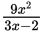is rewritten in the form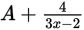, which of the following gives A in terms of x?

• A.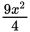• B.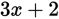• C.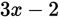• D.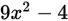Explanation:

B

Difficulty: Hard

Category: Passport to Advanced Math / Exponents

Strategic Advice: When a question involves equivalent expressions, compare how the original expression looks to how the desired result looks. Here, the original numerator has a higher power than the denominator, but the desired result doesn't. This is a clue that you need to divide.

Getting to the Answer: Set up the fraction as a long division problem and divide. Don't forget that anything left over at the end is the remainder and is written over the original denominator:Next, compare the result to the desired expression. Because the remainder 4 matches the numerator in the fraction part of the expression, A must be the quotient 3x + 2. Choice (B) is correct.